## Example Questions

### Example Question #1 : How To Find The Angle Of A Sector

John owns 8 black shirts, 7 red shirts, 6 blue shirts and 4 white shirts.  If he wants to make a circle chart of his shirts, what is the degree angle corresponding to the "blue shirt section?"

90 degrees

The answer cannot be determined from the above information.

25.0 degrees

86.4 degrees

43.2 degrees

86.4 degrees

Explanation:

We can set up a ratio to calculate the angle measure as such: 6/25 = x/360, since there are 360 degrees in a circle.  Solving, we obtain x = 86.4 degrees.

### Example Question #2 : Sectors

A circular pie is cut into 30 pieces. Two people wish to split a piece of the pie, but one person wants to have twice as much as the other person. What is the angle of the smaller piece produced in this manner?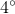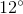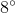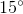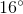Explanation:

First of all, calculate the angle of each of the full pieces of pie. This is easily found: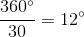Though small, this is what the information tells us! Now, we know that if two people are eating the piece, with one having twice the amount of the other, the angles must befor the smaller piece andfor the larger one. Thus, we can write the equation: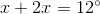Simplifying, we get: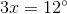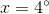That is a tiny piece, but that is what is called for by the data!

### Example Question #3 : Sectors

Quantity A: The angle of a circle's sector having an arc length of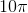and a radius of.

Quantity B: The angle of a circle's sector having an area of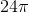and a radius of.

Which of the following relations is true?

Quantity B is larger than quantity A.

The relationship between the two values cannot be determined.

The two quantities are equal.

Quantity A is larger than quantity B.

Quantity A is larger than quantity B.

Explanation:

For each of these, you need to compute the total measurement applicable to the given data. For Quantity A, this will be the total circumference. For Quantity B, this will be the total area. You will then divide the given sector calculation by this total amount. By multiplying this percentage by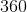, you will find the degree measure of each; however, you will merely need to stop at the percentage (since both are percentages of the same number, namely).

Quantity A

The total circumference is calculated using the standard equation:or, for our data: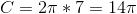Thus, our pertinent percentage is: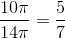Quantity B

For this, the area is computed by the formula:or, for our data: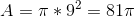Thus, our percentage is: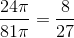Clearly,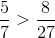, so A is larger than B.

Tired of practice problems?

Try live online GRE Math prep today.